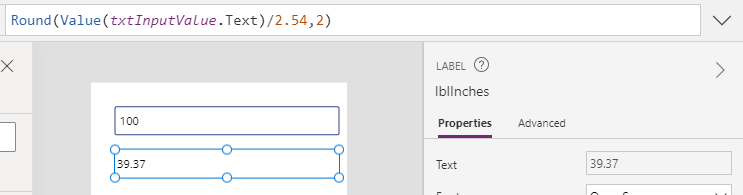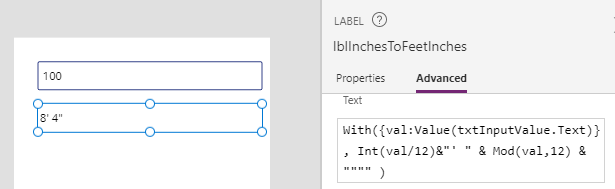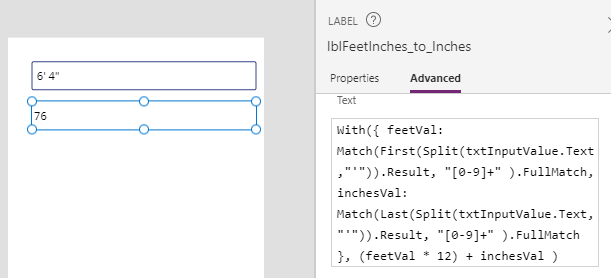# Formula - converting centimeters/meters to feet and inches, and vice versa

When building apps, particularly for audiences that include US and European users, there is often the requirement to convert measurements from centimeters/meters to feet and inches. This post covers the basic formula to carry out this task.

To demonstrate the basic formula that converts measurements from metric to Imperial, we'll create a screen with a text input control (called (txtInputValue).

The formula in this post describes the formula that we can add to a label to carry out the conversion.

## Converting centimeters/meters to inches

Starting with a simple example, we can convert centimetres to inches by dividing the input value by 2.54.

`Value(txtInputValue.Text)/2.54`
The screenshot below illustrates the output (100 centimeters = 39.37). Notice how we utilise the Round function to round the output to 2 decimal places.Since there are 100 cm in 1 m, we can convert metres to inches by dividing by 0.0254.
`Value(txtInputValue.Text)/0.0254`

## Converting inches to centimeters/meters

To convert inches to centimeters, we multiply the input value by 2.54.

`Value(txtInputValue.Text) * 2.54 `
To convert inches to meters, we multiply the input value by 0.0254
`Value(txtInputValue.Text) * 0.0254 `

## Converting meters to feet

To convert meters to feet, we multiply the input value by 3.2808.

`Value(txtInputValue.Text) * 3.2808 `

## Converting feet to meters

To convert feet to meters, we divide the input value by 3.2808.

`Value(txtInputValue.Text)/3.2808 `

## Converting inches to "feet and inches"

A common requirement is to produce output in feet and inches. Here's the basic formula to convert inches to feet and inches (in the format 8' 4").

`With({val:Value(txtInputValue.Text)},  Int(val/12)&"' " & Mod(val,12) & """")`## Converting centimeters/meters to "feet and inches"

As the natural progression of the previous example, we can convert centimeters/meters to feet and inches by including a conversion factor in our formula.

Here's the formula to convert centimetres to "feet and inches".

`With({val:(Value(txtInputValue.Text)/2.54)},  Int(val/12)&"' " & Mod(val,12) & """")`

Here's the formula to convert metres to "feet and inches".

`With({val:(Value(txtInputValue.Text)/0.0254)},  Int(val/12)&"' " & Mod(val,12) & """")`

## Converting "feet and inches" to inches

Given an input in "feet and inches" (in the format 5' 10"), a good starting point is to pass the string and to convert the input into inches. From here, we can use the earlier formulas to convert to centimeters or metres.

The formula beneath converts "feet and inches" into inches by splitting the input value by the single quote character. It uses a regular expression to return the numeric portion of both parts of the split output. The first part of the split output represents the feet value, and the last part represents the inches. We derive the final output by multiplying the feet value by 12 and adding the inches.

`With({        feetVal:           Match(First(Split(txtInputValue.Text,"'")).Result,                  "[0-9]+"           ).FullMatch,         inchesVal:           Match(Last(Split(txtInputValue.Text,"'")).Result,                  "[0-9]+"           ).FullMatch      },      (feetVal * 12) + inchesVal)`## Converting "feet and inches" to meters

The logical extension of the previous example is to convert "feet and inches" to meters. We can do this by multiplying the inches value by a conversion factor of 0.0254 like so:
`With({        feetVal:           Match(First(Split(txtInputValue.Text,"'")).Result,                  "[0-9]+"           ).FullMatch,         inchesVal:           Match(Last(Split(txtInputValue.Text,"'")).Result,                  "[0-9]+"           ).FullMatch      },      0.0254 * ((feetVal * 12) + inchesVal))`## Conclusion

When working with measurements, a common requirement is to convert meters/centimeters to feet/inches. This post summarised formulas to carry out this task.